Q:

# Longest Increasing Subsequence

Given a sequence A of size N, find the length of the longest increasing subsequence from the given sequence.

The longest increasing subsequence means to find a subsequence of a given sequence where the subsequence's elements are sorted in increasing order, and the subsequence is longest possible. This subsequence is not necessarily contiguous, or unique. Longest increasing subsequence is strictly increasing.

```    Input:
N=7
Sequence:
{2, 3, 4, 0, 1, 2, 3, 8, 6, 4}

Output:
Length of Longest increasing subsequence is 5
Longest increasing subsequence= {0, 1, 2, 3, 8} or {0, 1, 2, 3, 4}
```

Explanation with example

The possible increasing sub-sequences are,

Of Length 1 //each element itself is an increasing sequence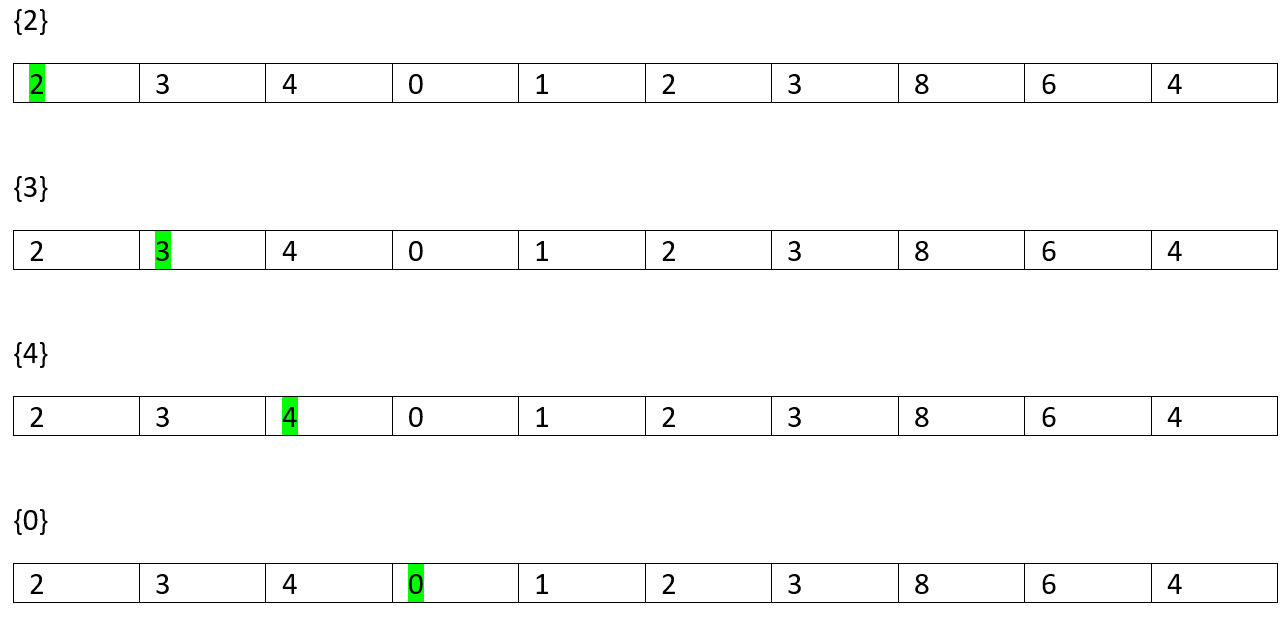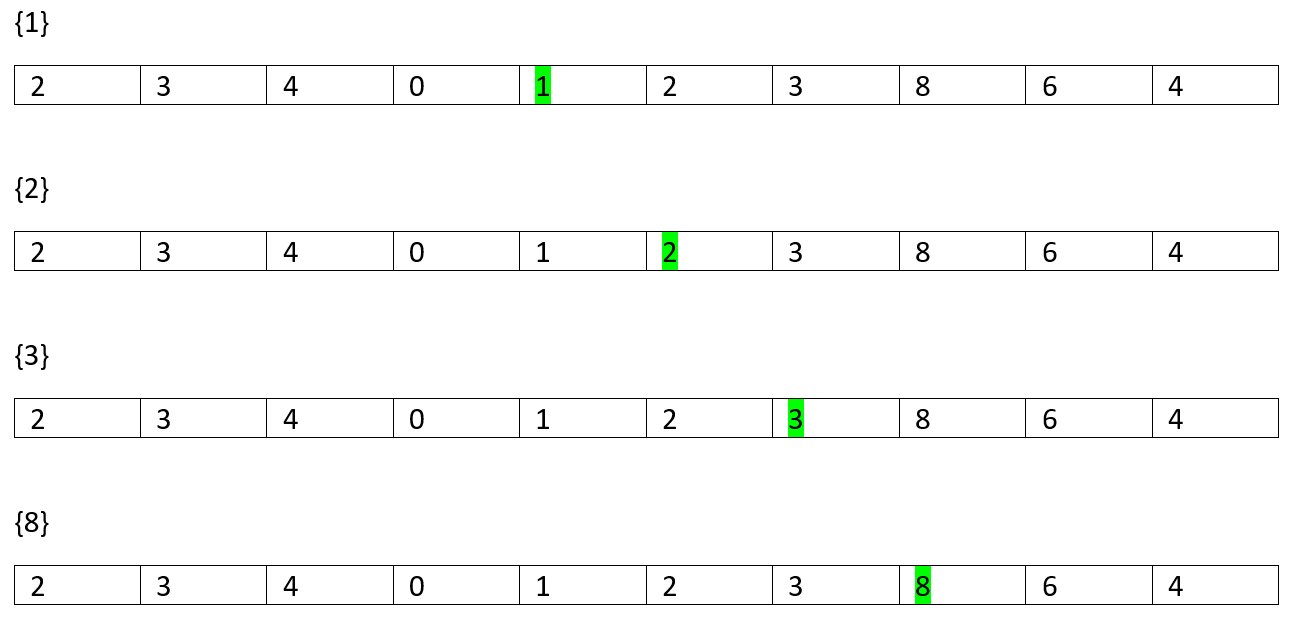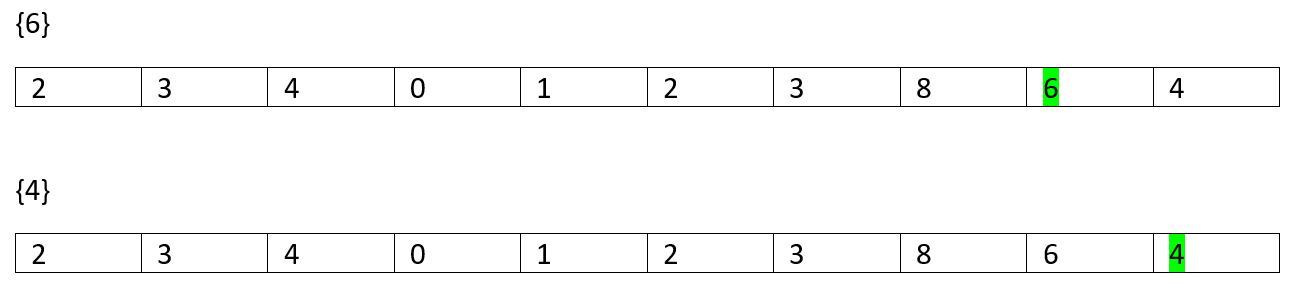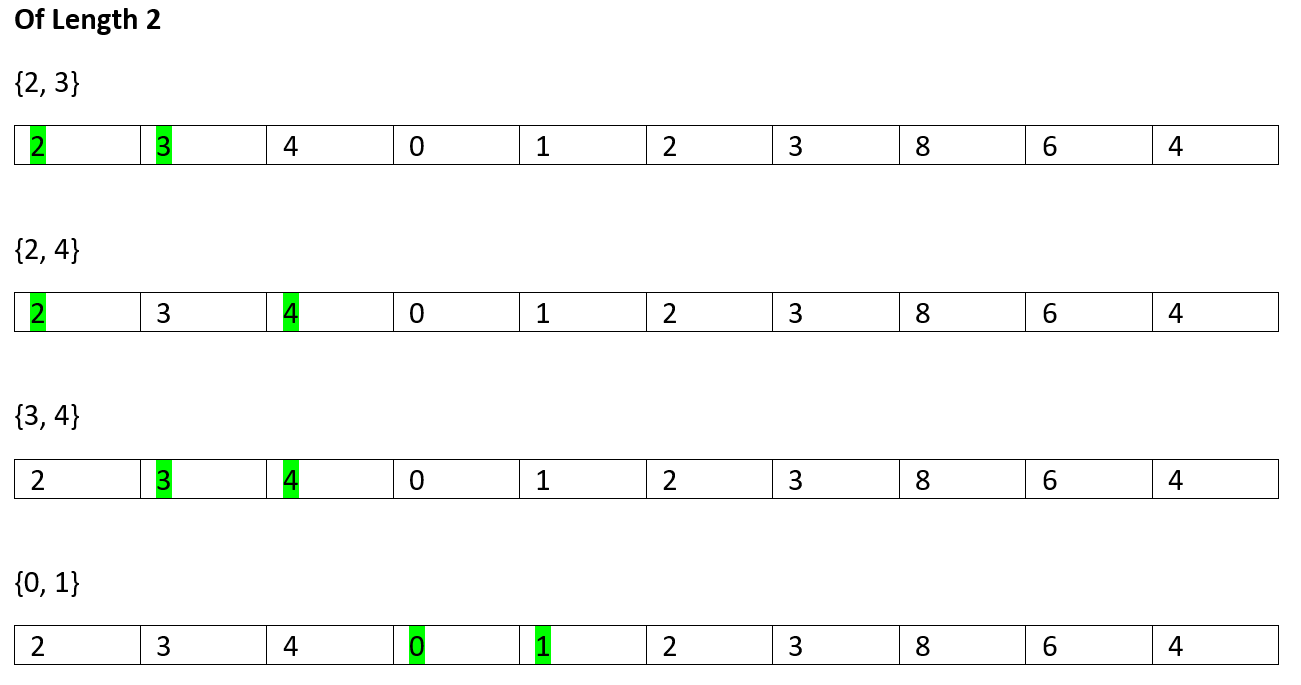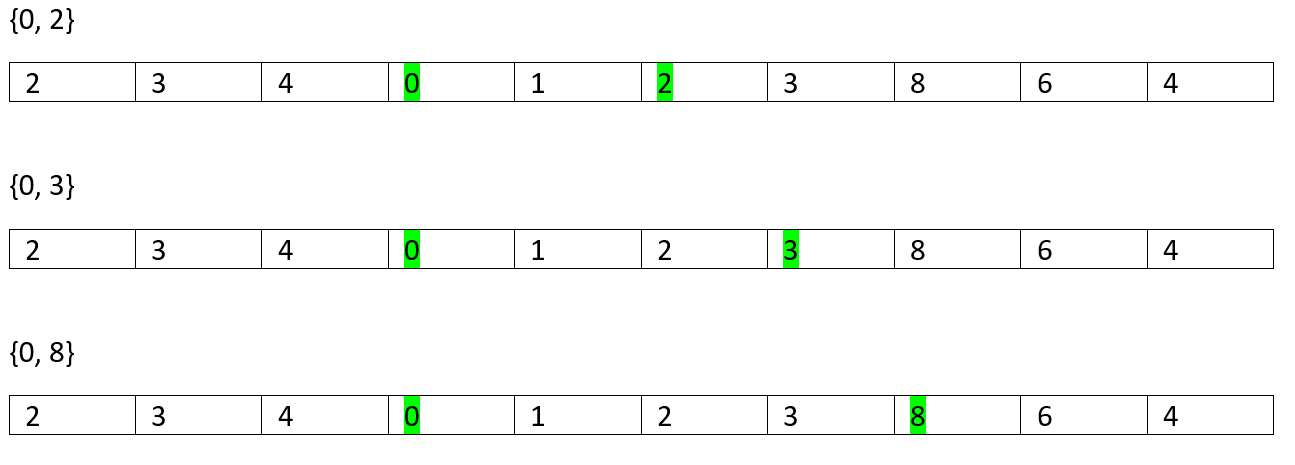So, on...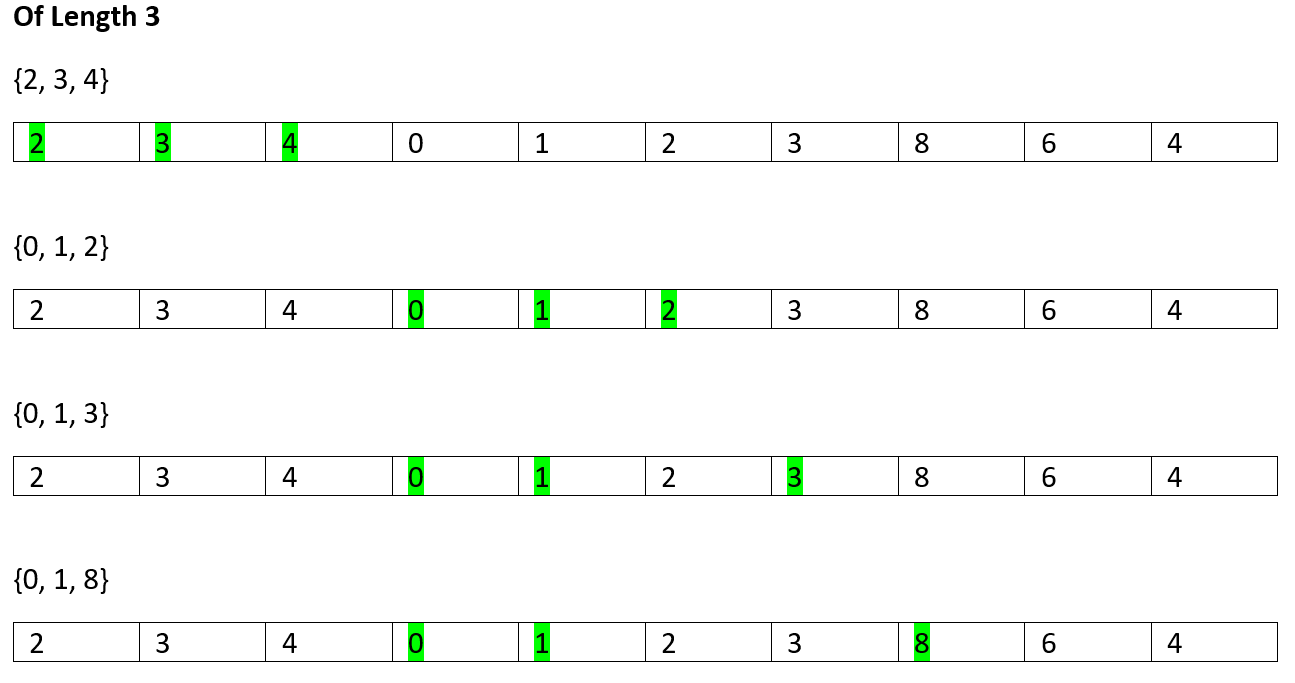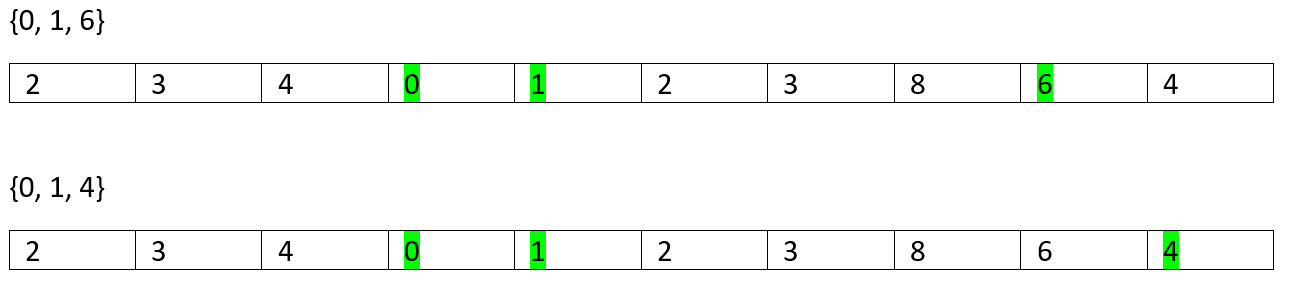So, on...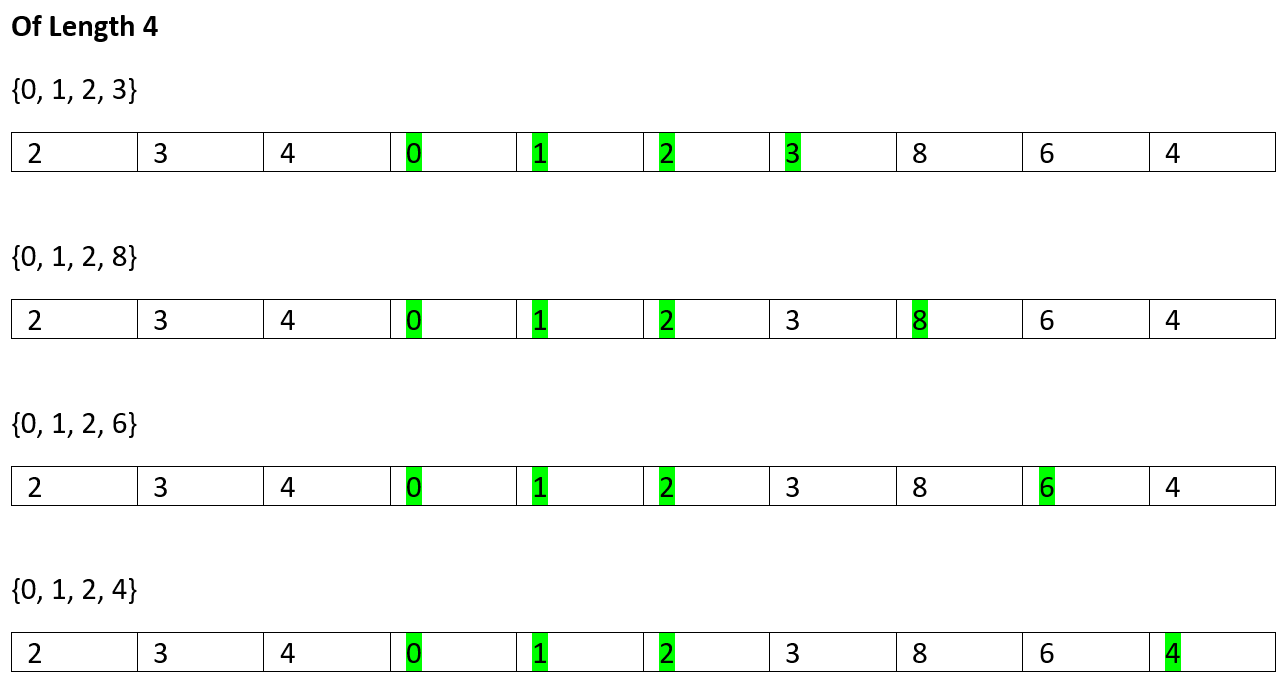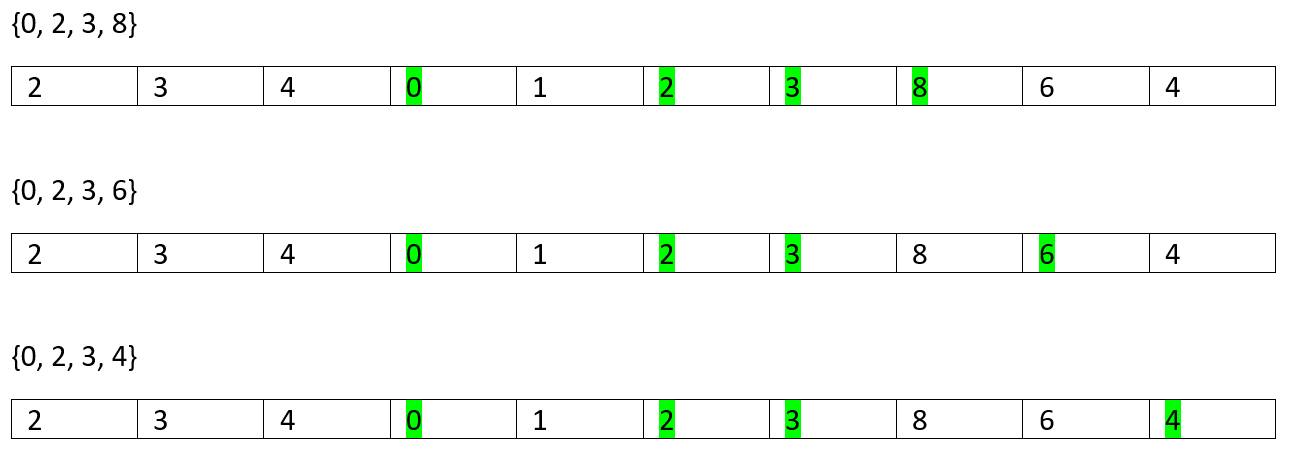So, on...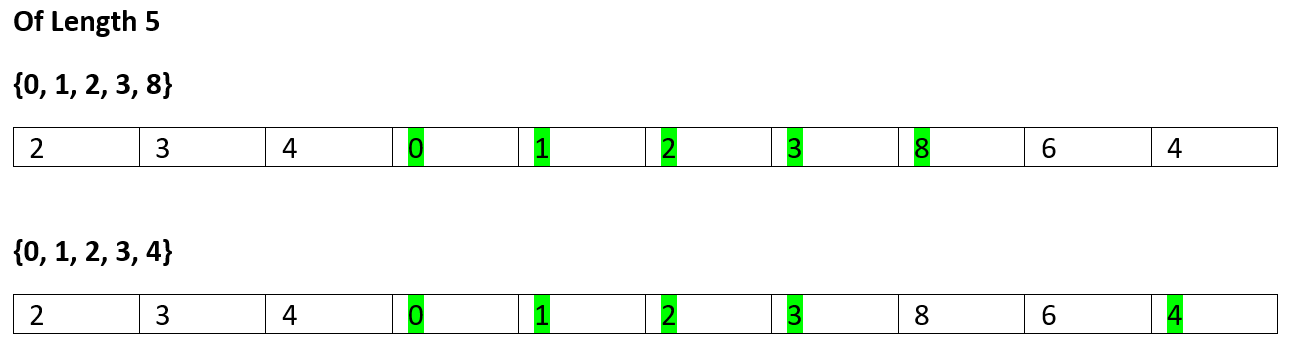No more
Of Length 6
None

So, the longest increasing subsequence length is 5.

Of course, in brute-force we can simply generate all increasing sequences and find the longest one. But it would take exponential time which is not a feasible solution. Hence, we choose Dynamic programming to solve.

We create a DP table to store longest increasing subsequence length.
It's intuitive that the minimum value would be 1 as each element represents the primitive sequence which is an increasing one.

So, the base value is 1.

Now,

```    Lis(i) = longest increasing subsequence starting from index 0 to index i
```

So,
To compute L(i) the recursion function is,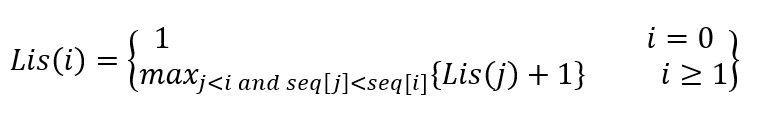As, the base value is 1, for every index iL(i) is at least 1.

```
1) Create the DP array, Lis[n]
2) Initialize the DP array.
for i=0 to n-1
lis[i]=1;
3) Now, to compute the Lis[i]
for index  i=1 to n-1
for previous index j=0 to i-1
// if (arr[i],arr[j]) is inceasing sequence
if(lis[i]<lis[j]+1 && a[i]>a[j])
lis[i]=lis[j]+1;
end for
end for
```

Initially DP table,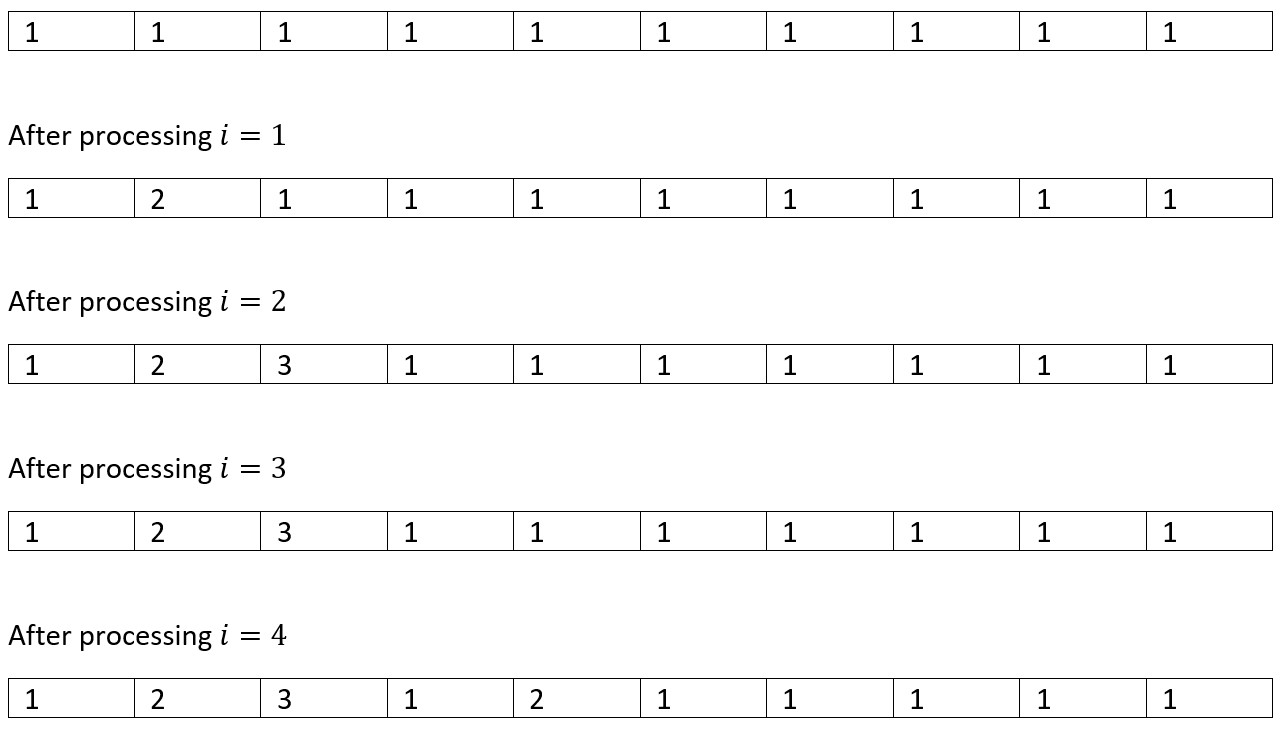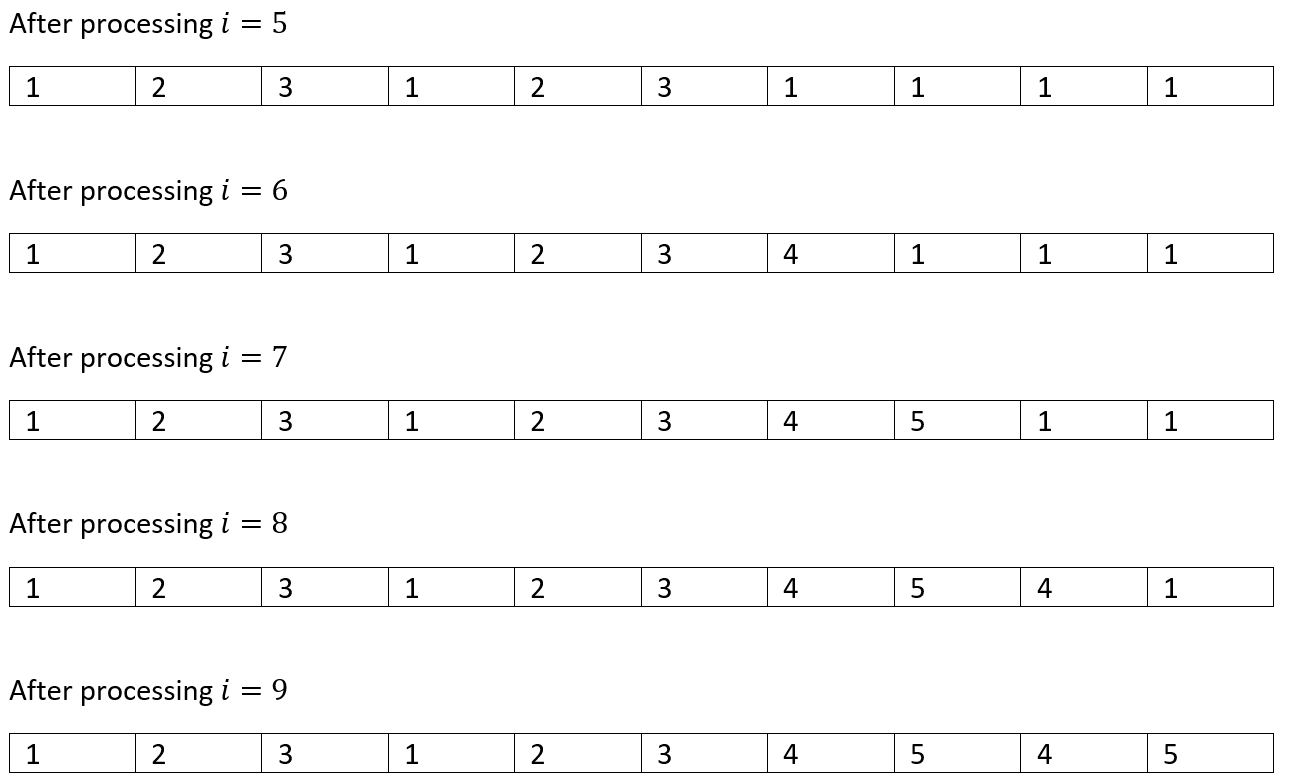So, the maximum out of this is 5
Hence, LIS=5.

C++ implementation:

``````#include <bits/stdc++.h>
using namespace std;

int max(int a, int b)
{
if (a > b)
return a;
else
return b;
}

int LIS(vector<int> a, int n)
{
int lis[n];

//base case
for (int i = 0; i < n; i++)
lis[i] = 1;

//fill up table
for (int i = 1; i < n; i++) {

for (int j = 0; j < i; j++) {
if (lis[i] < lis[j] + 1 && a[i] > a[j])
lis[i] = lis[j] + 1;
}
}
//return LIS
return *max_element(lis, lis + n);
}
int main()
{
int n, item;

cout << "Sequence size:\n";
scanf("%d", &n);
//input the array
vector<int> a;
cout << "Input sequence:\n";
for (int j = 0; j < n; j++) {
scanf("%d", &item);
a.push_back(item);
}

cout << "Length of longest incresing subsequence is: " << LIS(a, n) << endl;

return 0;
}``````

Output

```Sequence size:
10
Input sequence:
2 3 4 0 1 2 3 8 6 4
Length of longest incresing subsequence is: 5```